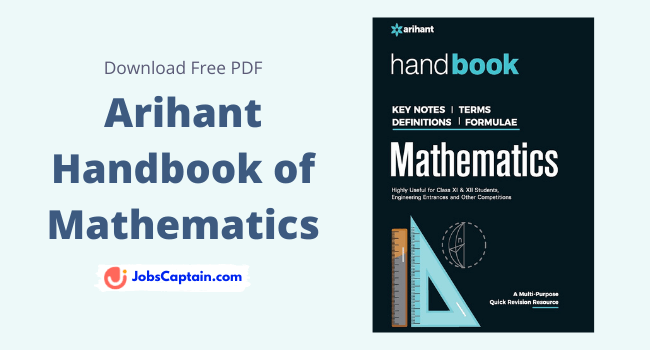By | March 24, 2022This Mathematics book covers nearly everything possible formulas, tables, values, maths keynotes, terms, definitions and many more.

This book explains all the Mathematics concepts very well and in a manner that every student will easy to understand.

Arihant Handbook of Mathematics PDF is very useful and handy when the exam is near. If you are JEE or NEET aspirant then you must have this book. It covers all maths topics of JEE and NEET. If you read it properly during your exam, it will 100% gives to good result.

For the engineering student, it is the best book forever to remember the formula.

 Book Name Mathematics Handbook Author Amit Rastogi Publication Arihant Publication Language English

## Book Contents

This book has all the basic definitions and formulas we need to know before entering into the concept. Arihant’s Mathematics Handbook covers all following topics:

1. Sets and Relations
2. Functions and Binary Options
3. Complex Numbers
4. Theory of Equations and Inequations
5. Sequences and Series
6. Permutations and Combinations
7. Binomial Theorem and Principle of Mathematical Induction
8. Matrix
9. Determinants
10. Probabilities
11. Trigonometric Functions, Identities and Equations
12. Solution of Triangles
13. Heights and Distance
14. Inverse Trigonometric Functions
15. Rectangular Axis
16. Straight Line
17. Circle
18. Parabola
19. Ellipse
20. Hyperbola
21. Limits, Continuity and Differentiability
22. Derivatives
23. Application of Derivatives
24. Indefinite Integrals
25. Definite Integrals
26. Applications of Integrals
27. Differential Equations
28. Vectors
29. Three Dimensional Geometry
30. Mathematical Reasoning
31. Statistics
32. Linear Programming Problem
33. Elementary Arithmetic – I
34. Elementary Arithmetic – II
35. Elementary Arithmetic – III
36. Elementary Algebra
37. Logarithms
38. Geometry
39. Mensuration
40. Some Great Mathematicians and their Contributions
Appendix I to VII

### Features of Book

1. Handy for revision of all formulae and concept
2. Good for quick revision (Easy for revision of formulas)
3. All arithmetic formulas of 11th and 12th are given in this book
4. Very helpful for all competitive examinations especially for UPSC, Bank PO, SSC CGL, CAT, Entrance Exams and also useful in 11th & 12th CBSC Exam

Mathematics Books:

Thank you for visiting our website. After reading this book, further, if you have any doubts about Mathematics then do comment below.

Category: Maths PDF